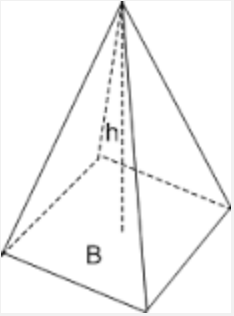# Area and Volume of a Pyramid

Instructions: Using this Area and Volume of a Pyramid calculator by entering the side $$l$$ and the height $$h$$ of a square pyramid and the units (cm, mt, ft, etc) and the solver will compute the corresponding surface area and volume of the given square pyramid.Type the side of the side of the base $$l$$ =Type the height of the pyramid $$h$$ =

This calculator deals with square pyramids (those pyramids with squared base), for which you need to provided the side $$l$$ of the base and the corresponding height $$h$$. A square pyramid looks like:### How to Compute the Area and Volume of a Pyramid?

In order to compute the surface area and volume of square pyramid with side $$l$$ and height $$h$$ we use the following formulas:

$\text{Area} = l^2 + 2l\sqrt{\frac{l^2}{4} + h^2}$ $\text{Volume} = \frac{l^2 h}{3}$

Computationally speaking, it is quite simple to compute the surface area and the volume of a square pyramid, by simply plugging the side $$l$$ and height $$h$$ in the above formulas. For example, if the side is $$l = 3$$ and the height is $$h = 4$$, we compute

$\text{Area} = l^2 + 2l\sqrt{\frac{l^2}{4} + h^2} = 3^2 + 2\cdot 3\sqrt{\frac{3^2}{4} + 4^2} = 34.632$ $\text{Volume} = \frac{l^2 h}{3} = \frac{3^2\cdot 4}{3} = 12$

which completes the calculation.

### Other geometric calculators

Our site provides a number of geometric calculators, including this sphere area and volume calculator , among many others.